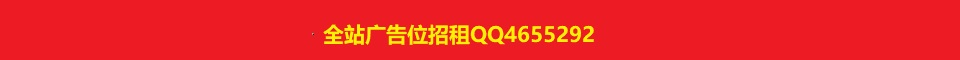黄金密度一般是多少？怎么测试黄金密度？1、用”k金”表示黄金的纯度

8k=8*4.166%=33.328%（333‰）
9k=9*4.166%=37.494%（375‰）
10k=10*4.166%=41.660%（417‰）
12k=12*4.166%=49.992%（500‰）
14k=14*4.166%=58.324%（583‰）
18k=18*4.166%=74.998%（750‰）
20k=20*4.166%=83.320%（833‰）
21k=21*4.166%=87.486%（875‰）
22k=22*4.166%=91.652%（916‰）
24k=24*4.166%=99.984%（999‰）
24k金常被认为是纯金，成为”1000‰”，但实际含金量为99.99%，折为23.988k。
2、用文字表达黄金的纯度

3、用分数表示黄金的纯度

4、用阿拉伯数字表示黄金的纯度

觉得文章有用就打赏一下文章作者

支付宝扫一扫打赏微信扫一扫打赏